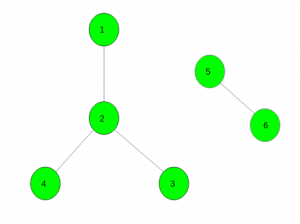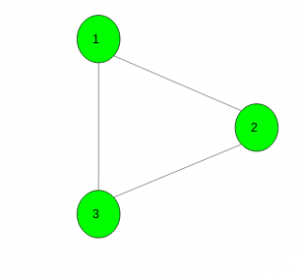# Find two disjoint good sets of vertices in a given graph

Given an undirected unweighted graph with N vertices and M edges. The task is to find two disjoint good sets of vertices. A set X is called good if for every edge UV in the graph at least one of the endpoint belongs to X(i.e, U or V or both U and V belongs to X).
If it is not possible to make such sets then print -1.

Examples:

Input :Output : {1 3 4 5} ,{2 6}
One disjoint good set contains vertices {1, 3, 4, 5} and other contains {2, 6}.

Input :Output : -1

Approach:
One of the observation is that there is no edge UV that U and V are in the same set.The two good sets form a bipartition of the graph, so the graph has to be bipartite. And being bipartite is also sufficient. Read about bipartition here.

Below is the implementation of the above approach :

## C++

 `// C++ program to find two disjoint ` `// good sets of vertices in a given graph ` `#include ` `using` `namespace` `std; ` `#define N 100005 ` ` `  `// For the graph ` `vector<``int``> gr[N], dis; ` `bool` `vis[N]; ` `int` `colour[N]; ` `bool` `bip; ` ` `  `// Function to add edge ` `void` `Add_edge(``int` `x, ``int` `y) ` `{ ` `    ``gr[x].push_back(y); ` `    ``gr[y].push_back(x); ` `} ` ` `  `// Bipartie function ` `void` `dfs(``int` `x, ``int` `col) ` `{ ` `    ``vis[x] = ``true``; ` `    ``colour[x] = col; ` ` `  `    ``// Check for child vertices ` `    ``for` `(``auto` `i : gr[x]) { ` ` `  `        ``// If it is not visited ` `        ``if` `(!vis[i]) ` `            ``dfs(i, col ^ 1); ` ` `  `        ``// If it is already visited ` `        ``else` `if` `(colour[i] == col) ` `            ``bip = ``false``; ` `    ``} ` `} ` ` `  `// Function to find two disjoint ` `// good sets of vertices in a given graph ` `void` `goodsets(``int` `n) ` `{ ` `    ``// Initially assume that graph is bipartie ` `    ``bip = ``true``; ` ` `  `    ``// For every unvisited vertex call dfs ` `    ``for` `(``int` `i = 1; i <= n; i++) ` `        ``if` `(!vis[i]) ` `            ``dfs(i, 0); ` ` `  `    ``// If graph is not bipartie ` `    ``if` `(!bip) ` `        ``cout << -1; ` `    ``else` `{ ` ` `  `        ``// Differentiate two sets ` `        ``for` `(``int` `i = 1; i <= n; i++) ` `            ``dis[colour[i]].push_back(i); ` ` `  `        ``// Print vertices belongs to both sets ` ` `  `        ``for` `(``int` `i = 0; i < 2; i++) { ` ` `  `            ``for` `(``int` `j = 0; j < dis[i].size(); j++) ` `                ``cout << dis[i][j] << ``" "``; ` `            ``cout << endl; ` `        ``} ` `    ``} ` `} ` ` `  `// Driver code ` `int` `main() ` `{ ` `    ``int` `n = 6, m = 4; ` ` `  `    ``// Add edges ` `    ``Add_edge(1, 2); ` `    ``Add_edge(2, 3); ` `    ``Add_edge(2, 4); ` `    ``Add_edge(5, 6); ` ` `  `    ``// Function call ` `    ``goodsets(n); ` `} `

## Java

 `// Java program to find two disjoint  ` `// good sets of vertices in a given graph  ` `import` `java.util.*; ` ` `  `class` `GFG  ` `{ ` ` `  `    ``static` `int` `N = ``100005``; ` ` `  `    ``// For the graph ` `    ``@SuppressWarnings``(``"unchecked"``) ` `    ``static` `Vector[] gr = ``new` `Vector[N],  ` `                            ``dis = ``new` `Vector[``2``]; ` `    ``static` `    ``{ ` `        ``for` `(``int` `i = ``0``; i < N; i++) ` `            ``gr[i] = ``new` `Vector<>(); ` `        ``for` `(``int` `i = ``0``; i < ``2``; i++) ` `            ``dis[i] = ``new` `Vector<>(); ` `    ``} ` `    ``static` `boolean``[] vis = ``new` `boolean``[N]; ` `    ``static` `int``[] color = ``new` `int``[N]; ` `    ``static` `boolean` `bip; ` ` `  `    ``// Function to add edge ` `    ``static` `void` `add_edge(``int` `x, ``int` `y) ` `    ``{ ` `        ``gr[x].add(y); ` `        ``gr[y].add(x); ` `    ``} ` ` `  `    ``// Bipartie function ` `    ``static` `void` `dfs(``int` `x, ``int` `col)  ` `    ``{ ` `        ``vis[x] = ``true``; ` `        ``color[x] = col; ` ` `  `        ``// Check for child vertices ` `        ``for` `(``int` `i : gr[x]) ` `        ``{ ` ` `  `            ``// If it is not visited ` `            ``if` `(!vis[i]) ` `                ``dfs(i, col ^ ``1``); ` ` `  `            ``// If it is already visited ` `            ``else` `if` `(color[i] == col) ` `                ``bip = ``false``; ` `        ``} ` `    ``} ` ` `  `    ``// Function to find two disjoint ` `    ``// good sets of vertices in a given graph ` `    ``static` `void` `goodsets(``int` `n) ` `    ``{ ` `        ``// Initially assume that graph is bipartie ` `        ``bip = ``true``; ` ` `  `        ``// For every unvisited vertex call dfs ` `        ``for` `(``int` `i = ``1``; i <= n; i++) ` `            ``if` `(!vis[i]) ` `                ``dfs(i, ``0``); ` ` `  `        ``// If graph is not bipartie ` `        ``if` `(!bip) ` `            ``System.out.println(-``1``); ` `        ``else` `        ``{ ` ` `  `            ``// Differentiate two sets ` `            ``for` `(``int` `i = ``1``; i <= n; i++) ` `                ``dis[color[i]].add(i); ` ` `  `            ``// Print vertices belongs to both sets ` ` `  `            ``for` `(``int` `i = ``0``; i < ``2``; i++) ` `            ``{ ` `                ``for` `(``int` `j = ``0``; j < dis[i].size(); j++) ` `                    ``System.out.print(dis[i].elementAt(j) + ``" "``); ` `                ``System.out.println(); ` `            ``} ` `        ``} ` `    ``} ` ` `  `    ``// Driver Code ` `    ``public` `static` `void` `main(String[] args) ` `    ``{ ` `        ``int` `n = ``6``, m = ``4``; ` ` `  `        ``// Add edges ` `        ``add_edge(``1``, ``2``); ` `        ``add_edge(``2``, ``3``); ` `        ``add_edge(``2``, ``4``); ` `        ``add_edge(``5``, ``6``); ` ` `  `        ``// Function call ` `        ``goodsets(n); ` `    ``} ` `} ` ` `  `// This code is contributed by ` `// sanjeev2552 `

## Python 3

 `# Python 3 program to find two disjoint ` `# good sets of vertices in a given graph ` `N ``=` `100005` ` `  `# For the graph ` `gr ``=` `[[] ``for` `i ``in` `range``(N)] ` `dis ``=` `[[] ``for` `i ``in` `range``(``2``)] ` `vis ``=` `[``False` `for` `i ``in` `range``(N)] ` `colour ``=` `[``0` `for` `i ``in` `range``(N)] ` `bip ``=` `0` ` `  `# Function to add edge ` `def` `Add_edge(x, y): ` `    ``gr[x].append(y) ` `    ``gr[y].append(x) ` ` `  `# Bipartie function ` `def` `dfs(x, col): ` `    ``vis[x] ``=` `True` `    ``colour[x] ``=` `col ` ` `  `    ``# Check for child vertices ` `    ``for` `i ``in` `gr[x]: ` `         `  `        ``# If it is not visited ` `        ``if` `(vis[i] ``=``=` `False``): ` `            ``dfs(i, col ^ ``1``) ` ` `  `        ``# If it is already visited ` `        ``elif` `(colour[i] ``=``=` `col): ` `            ``bip ``=` `False` ` `  `# Function to find two disjoint ` `# good sets of vertices in a given graph ` `def` `goodsets(n): ` `     `  `    ``# Initially assume that  ` `    ``# graph is bipartie ` `    ``bip ``=` `True` ` `  `    ``# For every unvisited vertex call dfs ` `    ``for` `i ``in` `range``(``1``, n ``+` `1``, ``1``): ` `        ``if` `(vis[i] ``=``=` `False``): ` `            ``dfs(i, ``0``) ` ` `  `    ``# If graph is not bipartie ` `    ``if` `(bip ``=``=` `0``): ` `        ``print``(``-``1``) ` `    ``else``: ` `         `  `        ``# Differentiate two sets ` `        ``for` `i ``in` `range``(``1``, n ``+` `1``, ``1``): ` `            ``dis[colour[i]].append(i) ` ` `  `        ``# Print vertices belongs to both sets ` `        ``for` `i ``in` `range``(``2``): ` `            ``for` `j ``in` `range``(``len``(dis[i])): ` `                ``print``(dis[i][j], end ``=` `" "``)  ` `            ``print``(``'\n'``, end ``=` `"") ` ` `  `# Driver code ` `if` `__name__ ``=``=` `'__main__'``: ` `    ``n ``=` `6` `    ``m ``=` `4` ` `  `    ``# Add edges ` `    ``Add_edge(``1``, ``2``) ` `    ``Add_edge(``2``, ``3``) ` `    ``Add_edge(``2``, ``4``) ` `    ``Add_edge(``5``, ``6``) ` ` `  `    ``# Function call ` `    ``goodsets(n) ` ` `  `# This code is contributed ` `# by Surendra_Gangwar `

Output:

```1 3 4 5
2 6
```My Personal Notes arrow_drop_upCheck out this Author's contributed articles.

If you like GeeksforGeeks and would like to contribute, you can also write an article using contribute.geeksforgeeks.org or mail your article to contribute@geeksforgeeks.org. See your article appearing on the GeeksforGeeks main page and help other Geeks.

Please Improve this article if you find anything incorrect by clicking on the "Improve Article" button below.